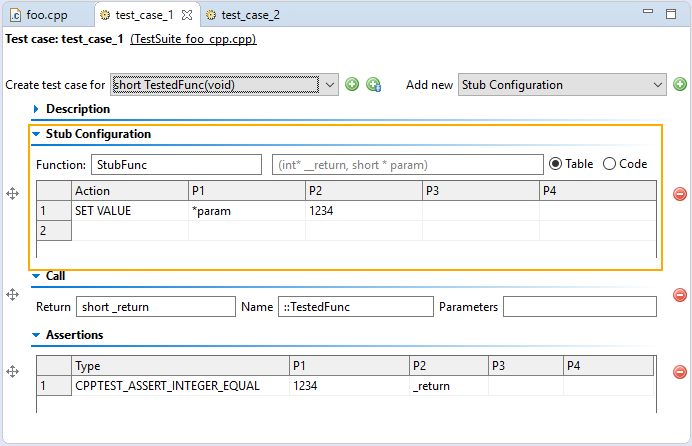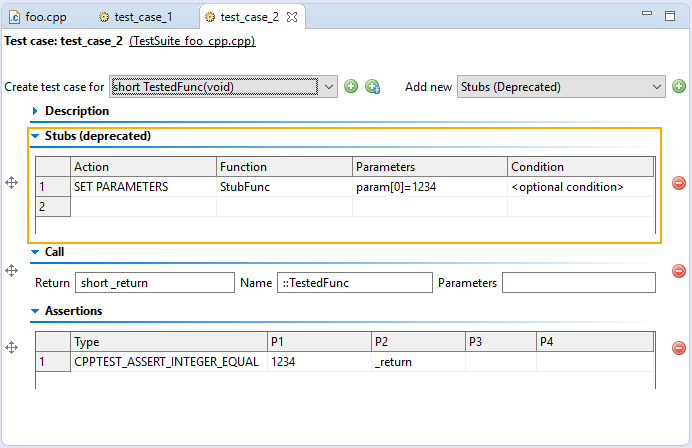# Modify value of output parameter in Test Case Editor ??

Hello,
I have a stubbed function like this :
int StubFunc(short * param)
(Which is supposed to store a value at the address pointed to by param, so, an output parameter)
In order to correctly cover my code, I need to be able to modify the value of param when a stub call is made like the original function would do. However I can't find any syntax that works in the stub box of the Test Case Editor. I can modify the return value, but if I try anything other than "param=[some int]" (which does nothing), the test case fail. What's the correct syntax to modify the value of output parameters in the Test Case Editor ??
Thanks.

Tagged:

## Comments

• If I understand your case correctly you should be using the following syntax:

*param = [some int];

For example:
*param = 22;

Let me know if it helps.

• To visualize @Mirek's comment:

1. Assuming you have C++test 10.4.x:2. If you're still on C++test 10.3.x:• Thanks to you both ! I had tried *param=1234 on the 10.3, I actually switched to the 10.4 yesterday in which it worked.

Sign In or Register to comment.